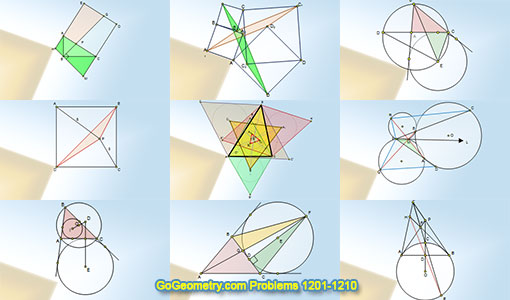Geometry Problems 1201-1210. Level: High School, CollegeGeometry Problems 1201 - 1210Geometry Problem 1201 Triangle, Interior Perpendicular Bisector, Midpoint, Area. Geometry Problem 1202 Four Squares, Two Congruent Parallelograms, Congruence. Geometry Problem 1203 Right Triangle, Square, Angle Bisector, Three Congruent Quadrilaterals. Geometry Problem 1204 Square, Triangle, Area. Geometry Problem 1205 Triangle, Centroid, Outer and Inner Napoleon Equilateral Triangles. Geometry Problem 1206 Circle, Angle Bisector, Secant, Triangle, Circumcircle, Congruence, Area. Geometry Problem 1207 Triangle, Circle, Incenter, Circumcenter, Excenter, Circumradius, Perpendicular, 90 Degrees. Geometry Problem 1208 Triangle, Circle, Excircle, Excenter, Diameter, Perpendicular, 90 Degrees, Equal Areas. Geometry Problem 1209 Triangle, Circle, Excircle, Excenter, Circumcircle, Congruence. Geometry Problem 1210 Circle, Tangent Line, Secant, Chord, Collinear Points.

Home | Sitemap | Search | Geometry | Problems | Visual Index | 10 ProblemsEmail | Post a comment | by Antonio Gutierrez
Last updated: Feb 1, 2017